Synergetics. Etudes 70.

Is Dedicated to Centennial of Burthday of Outstanding Scientist, Professor

Basin Abram M.

Basina G. I., Basin M. A.

SIC ”Synergetics” of Saint-Petersburg Association of Scientist and Scholars.

Etude 2.

Exponents of Circumference as Phase Curves of Nonlinear Dynamically Systems

20 November 2009

At the investigation of dynamically systems for the determination of their integral parameters we recommend the introduction of complex parameter of wholeness and also finding and decision of complex differential equation, to which it satisfies.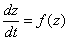,                                                               (1)

where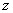may be complex algebraic variable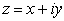or complex spiral variablle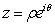. Phase trajectory which is the decision of equation (1), may be written in the form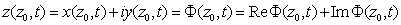,                     (2)

where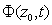is the meaning of algebraic complex coordinate of phase trajectory, passing through the point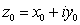at the moment of time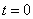. The same decision may be written and in spiral varieties.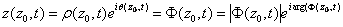.                          (3)

Shall we introduce new function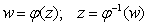.

Then the equation (1) will transform in the form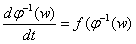, or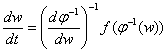.                                                 (4)

Corresponding phase trajectory for variable quantity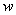has the form: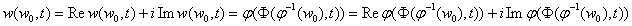So, having the decision of one equation (1), we may build the class of derivative nonlinear differential equations, connected with given equation, which decisions are building on the base of decision of given equation. It is interesting one important particular case of fulfilled transformations. As the main equation we  take the linear complex differential equation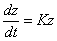.                                                                    (5)

Its decision may be received with such a matter.: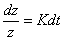. Integrating both parts of received equation, we shall receive: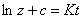. Exhibiting both parts of received equation, we receive: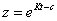. If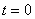, then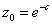, and phase trajectories of decision of this equation have the form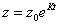.                                                                (6).

Shall we represent: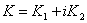. Then the decision of the equation (5) shall receive the form: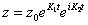.                                                            (7)

Phase curves represent the developing or twisting spirals, or coming to zero, or going away on the infinity. Further us will be interesting the particular case, corresponding to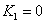. In this case we have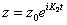. If we shall consider, not losing the generality, that the primary meaning of the unknown quality is the real number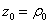, then the equation of phase trajectory has the form: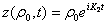.                                                        (8)

The point of phase trajectory of the equation (5) in considerable particular case moves on the circumference with radius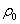- on the cyclic trajectory. Further shall we take that the connection between complex variablesandhas the form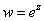(9).

Then the equation, describing the dynamics of variablereceives the form: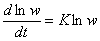, or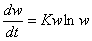.                                                       (10)

This equation we met at the investigation of dynamics of populations.

Cyclic phase trajectories of dynamically system, describing with this equation, have the form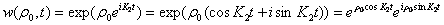The last equality describes the trajectory of the point, moving on the exponent of circumference (See Etude №1). So, the exponent of circumference is a cyclic phase trajectory of any dynamically system, describing with complex differential equation (10).

But with this not exhaust the class of differential equations, having phase trajectories with the type of exponent of circumference. It is known that in dynamically systems of different type occurs the bifurcation of cycles birth. If we shall consider the variables of these systems as the logarithms of any new variables, then for these new variables we receive the equations, for which the bifurcation of cycles birth shall transform in the bifurcation of the birth of any new trajectory which is the exponent of circumference.

The undergoing from cyclic phase trajectory to the phase trajectory in the form of the exponent of circumference must be standard for self organizing information- transport systems, which have different but strongly connected dimensions of variables, describing them.